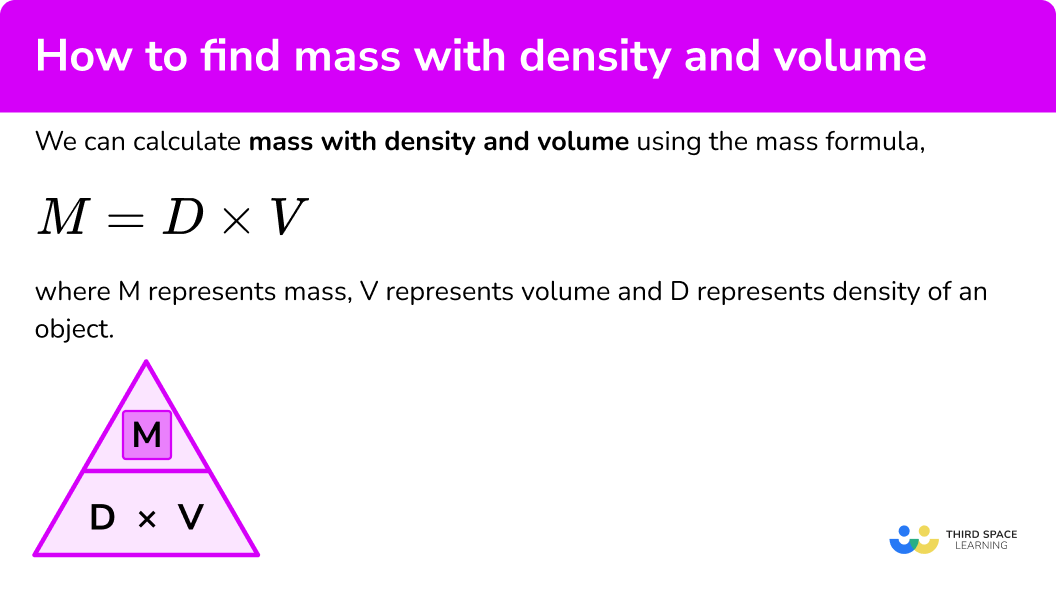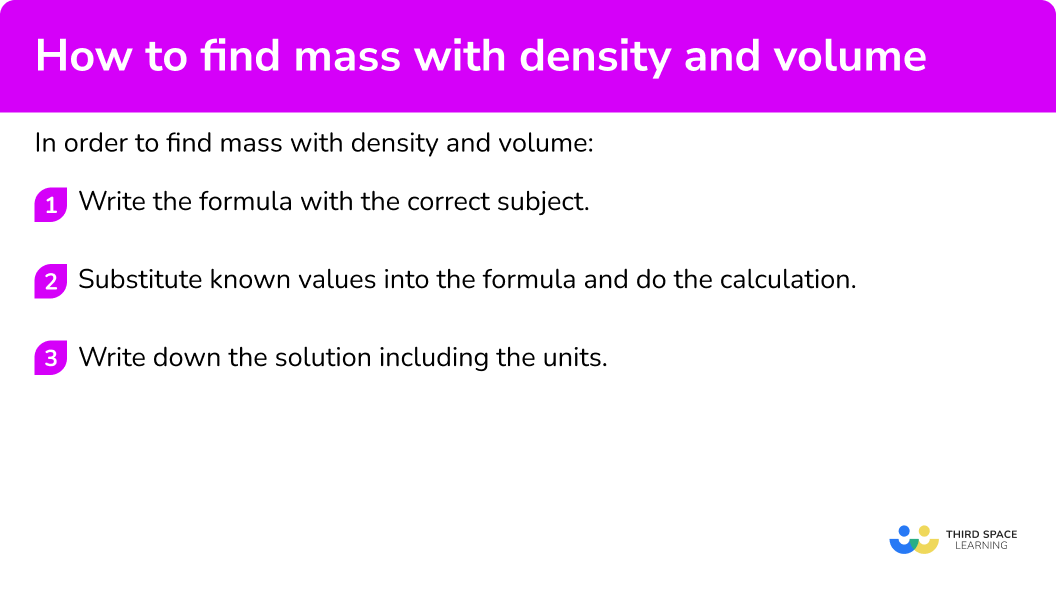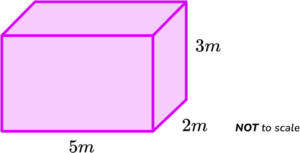GCSE Maths Ratio and Proportion Compound Measures Mass Density Volume

How To Find Mass With Density And Volume

# How To Find Mass With Density And Volume

Here we will learn about how to find mass with density and volume, including using the density formula.

There are also how to find mass with density and volume worksheets based on Edexcel, AQA and OCR exam questions, along with further guidance on where to go next if you’re still stuck.

## What is mass (with density and volume)?

We can calculate how to find mass with density and volume by using the mass formula,

M=D\times{V}

where \textbf{M} represents mass, \textbf{V} represents volume and \textbf{D} represents density of an object.

This is the density equation or density formula, although to calculate the mass, we have rearranged the formula to make the mass the subject. We can also represent this using the density mass volume triangle,

### What is mass (with density and volume)?### ● Mass

The mass of an object is the amount of matter there is in an object.

The standard SI units of mass is kilograms (kg) but we can also use other metric units such as grams, milligrams or tonnes. The following table shows the conversion of 1kg to these other units.

Unit \bf{1kg \ =}
Tonne (t) 0.001 \ t
Grams (g) 1000 \ g
Milligrams (mg) 1,000,000 \ mg

Imperial units include stones (st), pounds (lb), ounces (oz) and imperial tons (ton).

The imperial ton is not the same as the metric tonne, as 1 \ ton = 1.01605 \ t.

Mass calculator

The mass of an object can be calculated if we know the density and volume of the object.

Mass is directly proportional to the volume of an object with a constant density; the heavier the mass, the larger the volume when the density remains the same.

This means that we can state M\propto{V} or M=kV where the constant of proportionality, k, represents the density of the object.

### ● Volume

The volume of an object is how much space an object or substance takes up. The SI unit for volume is cubic metres written as m^3. Other volumetrics we should be aware of are the cubic centimetre (cm^{3}) and the cubic millimetre (mm^{3}).

The volume of a liquid is referred to as its capacity where 1 \ cm^3 = 1 \ ml \ (1 millilitre), and hence 1000 \ cm^3 = 1L (1 litre).

Volume calculator

The volume of a substance can be calculated if we know the density and mass of the object.

Volume is inversely proportional to the density of an object with a constant mass; the greater the volume, the lower the density of the object or substance with a constant mass.

This means that, for volume V and density D, we can state V\propto\frac{1}{D} or V=\frac{k}{D} where the constant of proportionality, k, represents the mass of the object.

### ● Density

Density is another physical property of an object or substance. The density of a material is a compound measure that relates how much matter there is in the substance per unit volume.

For example, a sponge has a higher density when it is squeezed as its volume has decreased, but the mass has not changed. Conversely, when the sponge has been released, it has a lower density as the volume has increased but the mass has stayed the same.

Below is a table describing some density measurements.

UnitDescription
g/cm^3 Grams per cubic centimetre
kg/m^3 Kilograms per cubic metre
mg/ml Milligrams per millilitre

Notice that the first unit of each density unit is a mass (grams, kilograms, milligrams) and the divisor is a volume measurement (cubic centimetres, cubic metres, and millilitres).

Remember: 1cm^3 = 1 \ ml.

At 4^{o}C, the density of water is 1000 \ kg/m^{3}, which is the same as 1g/cm^{3}.

Density calculator

The density of a substance can be calculated if we know the volume and mass of the object.

Density is inversely proportional to the volume of an object with a constant mass; the greater the density, the lower the volume of the object or substance with a constant mass.

This means that, for density D and volume V, we can state D\propto\frac{1}{V} or D=\frac{k}{V} where the constant of proportionality, k, represents the mass of the object.

## How to find mass with density and volume

In order to find mass with density and volume:

1. Write the formula with the correct subject.
2. Substitute known values into the formula and do the calculation.
3. Write down the solution including the units.

### Explain how to find mass with density and volume### Related lessons oncompound measures

How to find mass with density and volume is part of our series of lessons to support revision on compound measures. You may find it helpful to start with the main compound measures lesson for a summary of what to expect, or use the step by step guides below for further detail on individual topics. Other lessons in this series include:

## How to find mass with density and volume examples

### Example 1: calculate mass in grams

Find the mass of an object with volume 300 \ cm^{3} and density 5 \ g/cm^{3}. Give your answer in grams.

1. Write the formula with the correct subject.

M=DV

2Substitute known values into the formula and do the calculation.

\text{Mass}=\text{density}\times \text{volume}=5\times 300=1500

3Write down the solution including the units.

Mass = 1500g

### Example 2: calculate mass in grams

Find the mass of an object with volume 450 \ cm^3 and density of the material is 7.2 \ g/cm^{3}. Give your answer in grams.

M={D}\times{V}

M={D}\times{V}=7.2\times{450}=3240

Mass = 3240g

### Example 3: calculate mass in kilograms

Find the mass of a solid object with volume 4 \ m^3 and density of the material is 7500 \ kg/m^{3}. Give your answer in tonnes.

M={D}\times{V}

M={D}\times{V}={7500}\times{4}=30,000

As 1 \ tonne = 1000 \ kg, we need to divide 30,000 by 1000 to convert the units from kilograms to tonnes.

30000\div{1000}=30

Mass = 30 \ t

### Example 4: calculate mass when units need converting

Find the mass of an object with volume 950 \ cm^3 and density of the material is 18.2 \ g/cm^{3}. Write your answer in kilograms.

M={D}\times{V}

M={D}\times{V}={18.2}\times{950}=17290

The mass is currently in grams, and we need to convert it to kilograms.

We do this by dividing the mass by 1000 as 1000g = 1 \ kg.

17290\div{1000}=17.29

Mass = 17.29 \ kg

### Example 5: calculate mass when units need converting

Find the mass of an object with volume 0.000875 \ m^3 and density 15.3 \ g/cm^{3}. Write your answer in kilograms.

M={D}\times{V}

As the volume unit is in m^3 and the density unit is in g/cm^3 we need to convert the volume to cm^{3}. As 1m^3 = 1,000,000 \ cm^{3} , we need to multiply the volume by 1000000 \ (= 1 million, or 10^{6}) first, then substitute the values into the formula.

V=0.000875\times{1000000}=875cm^{3}

Now V=875 \ cm^3 and D=15.3 \ g/cm^3 and so

M={D}\times{V}={15.3}\times{875}=13387.5 .

Mass = 13.3875 \ kg

### Example 6: calculate mass when units in standard form

Find the mass of an object with volume (2 \times 10^{6} ) \ cm^3 and density of the material is 3 100 \ kg/m^{3}. Write your answer in tonnes.

M={D}\times{V}

The volume is currently in cubic centimetres, which we can convert to cubic metres by dividing by 1000000.

(2\times{10}^{6})\div{1000000}=2\times{10^{6}}\div{10^{6}}=2

Now V=2m^3 and so

M={D}\times{V}={3100}\times{2}=6200 .

The answer is required in tonnes and so dividing 6200 by 1000, we have

Mass = 6.2 \ t.

### Common misconceptions

• Incorrect orientation of the formula

It is common to write the relationship between density, mass, and volume incorrectly. A useful way to remember the correct orientation is using the units for density. As density is a mass, divided by a volume, if we multiply the density by a volume, the units we are left with are a mass.

• Units of measure

We tend to use metric units for mass, density and volume. We need to be able to convert between metric units such as knowing there are 100 \ cm in 1 metre. Occasionally you might come across other types of units such as imperial units like the cubic foot.

• Converting units (linear)

You may have worked out grams, but the answer needs to be in kilograms.

Remember that 1 \ kg = 1000 \ g.

Remember that 1 \ tonne = 1000 \ kg.

• Converting units (volume)

A volume uses cubic units such as m^3 and cm^{3}, or capacity units such as ml and L. The conversion for cubic units is the cube of the scale factor. For example, a cube with a side length of 1m has a volume of 1m^{3}.

The same cube has a side length of 100 \ cm, and so the volume of the cube is 100^3 = 1,000,000 \ cm^{3}. This means that 1 \ m^{3}=1,000,000 \ cm^3 and so if we convert cubic metres to cubic centimetres, we need to multiply the volume by 1,000,000.

### Practice how to find mass with density and volume questions

1. The volume of an object is 120 \ cm^{3}. The density of the material the object is made from is 3 \ g/cm^{3}. Calculate the mass of the object in grams.

400 \ g360 \ g40 \ g36 \ g\text{Mass}=\text{density}\times \text{volume}=3\times 120=360

2. The volume of an object is 170 \ cm^{3}. The density of the material the object is made from is 4 \ g/cm^{3}. Calculate the mass of the object in grams.

620 \ g660 \ g640 \ g680 \ g\text{Mass}=\text{density}\times \text{volume}=4\times 170=680

3. The volume of an object is 3 \ m^{3}. The density of the material the object is made from is 6 \ 000 \ kg/m^{3}. Calculate the mass of the object in tonnes.

1.8 \ kg0.18 \ t18 \ t1.8 \ t\begin{aligned} &\text{Mass}=\text{density}\times \text{volume}=6000\times{3}=18 000 \\\\ &18000\div{1000}=18 \ t \end{aligned}

4. The volume of an object is 0.00082 \ m^{3}. The density of the material the object is made from is 13 \ g/cm^{3}. Calculate the mass of the object in kilograms.

10 \ 660 \ g1 \ 066 \ g1.066 \ kg10.66 \ kg\begin{aligned} &0.00082 \ m^3 = 820 \ cm^3 \\\\ &\text{Mass}=\text{density}\times \text{volume}=13\times 820=10 660 \end{aligned}

As 1 \ kg = 1000 \ g, we need to divide by 1000 to convert to kilograms.

10 \ 660 \div 1000 = 10.66 \ kg

5. The volume of an object is 4.5 \ m^{3}. The density of the material the object is made from is 2700 \ kg/m^{3}. Calculate the mass of the object in tonnes.

12150 \ tonnes121.5 \ tonnes0.1215 \ tonnes12.15 \ tonnes\text{Mass}=\text{density}\times \text{volume}=2700\times 4.5=12 150

We need to divide by 1000 to convert to tonnes.

12150\div{1000}=12.15 \ tonnes

6. The volume of an object is 5.9 \ m^{3}. The density of the material the object is made from is 63 \ g/cm^{3}. Calculate the mass of the object in tonnes.

37.17 \ tonnes371.7 \ tonnes371700000 \ g0.003717 \ tonnes\begin{aligned} &5.9 \ m^{3}=5900000 \ cm^{3} \\\\ &M=D\times{V}=63\times{5900000}=371700000 \\\\ &1 \ t=1000 \ kg=1000000 \ g \\\\ &371700000\div{1000000}=371.7 \ t \end{aligned}

### How to find mass with density and volume GCSE questions

1. A block of wood had density 1.6 \ g/cm^3 and a volume of 300 \ cm^{3}.

Calculate the mass of the block of wood.

(2 marks)

1.6\times \ 300

(1)

480

(1)

2. A platinum bar has a density of 21.4 \ g/cm^3 and a volume of 480 \ cm^{3}.

A gold bar has a density of 19.32 \ g/cm^3 and a volume of 530 \ cm^{3}.

Which bar has the greater mass?

(3 marks)

21.4\times 480=10272 \ g

(1)

19.32\times 530=10239.6 \ g

(1)

Platinum

(1)

3. Here is a block of iron.It measures 5 \ m by 2 \ m by 3 \ m.

The density of iron is 7800 \ kg/m^{3}.

A crane has a maximum load weight of 2.4 \times 10^{6} \ N.

Given that w=mg where w is the weight in Newtons, g=9.807 \ m/s^{2}, and m is the mass in kilograms, can the crane pick up the block of iron?

(5 marks)

5\times 2\times 3=30 \ m^3

(1)

7800\times 30=234 000 / kg

(1)

234000\times{9.807}=2294838

(1)

2294838=2.294838\times{10}^{6} \ N\textbf{ or }2.4\times{10}^{6}=2400000 \ N

(1)

Yes

(1)

## Learning checklist

You have now learned how to:

• Use compound units such as density to solve problems

## Still stuck?

Prepare your KS4 students for maths GCSEs success with Third Space Learning. Weekly online one to one GCSE maths revision lessons delivered by expert maths tutors.

Find out more about our GCSE maths tuition programme.